Not all businesses hold assets for sale. They create an asset management plan to effectively and efficiently handle their properties. Some of them, such as properties, buildings, vehicles, pieces of equipment, machinery, and other fixed assets, are bought for daily operating activities over a certain period of time. A depreciation schedule is a chart or table in which businesses or organizations can efficiently track their depreciation expenses with expenses sheets on each asset over the years. Depreciation is a term used to describe how much value an asset has been used up or a method that distributes the cost of a particular fixed asset over its anticipated useful life.

## 1. Depreciation Entry Worksheet Template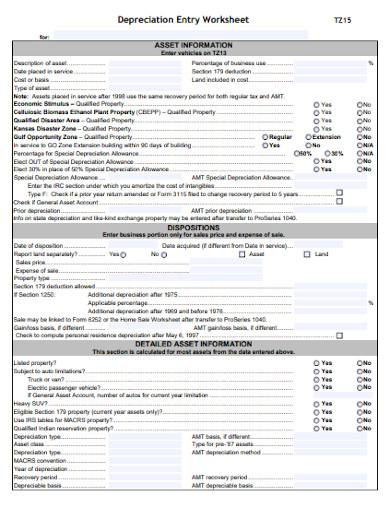inthezoneonline.com

## 2. Income Tax Depreciation Adjustment Worksheet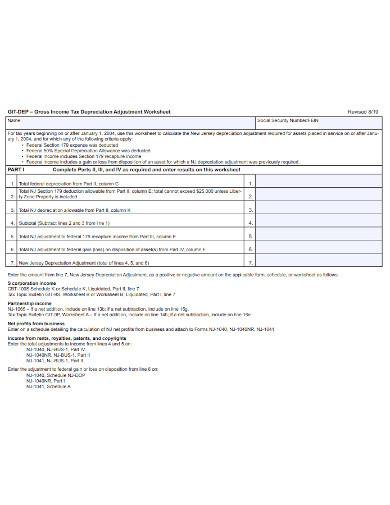state.nj.us

## 3. Rental Property Depreciation Worksheet Template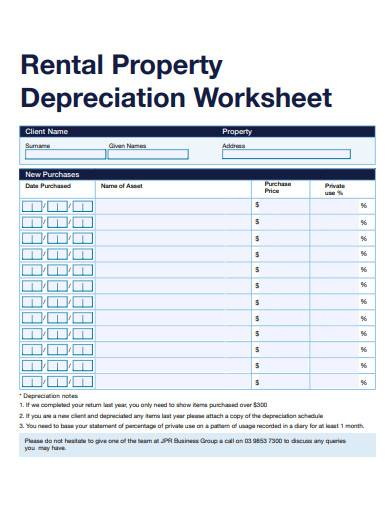static1.squarespace.com

## 4. Depreciation Detail Listing Worksheet Template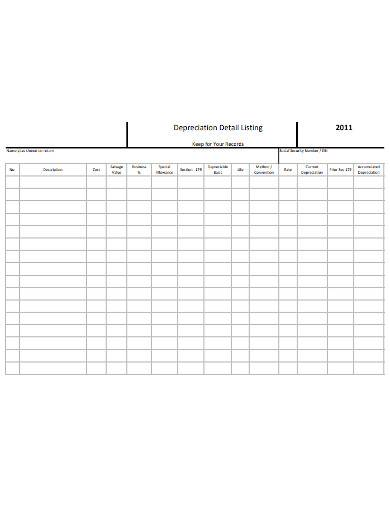## 5. Tax Depreciation Worksheet Template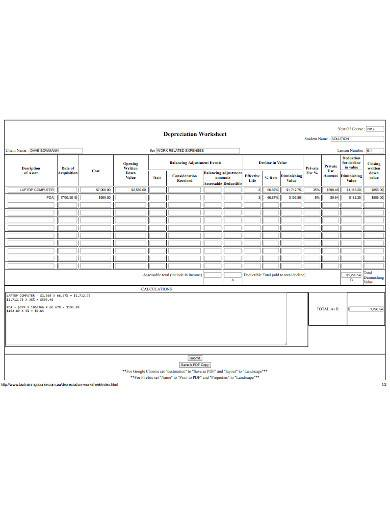q-tax.com.au

## 6. Depreciation and Amortization Worksheet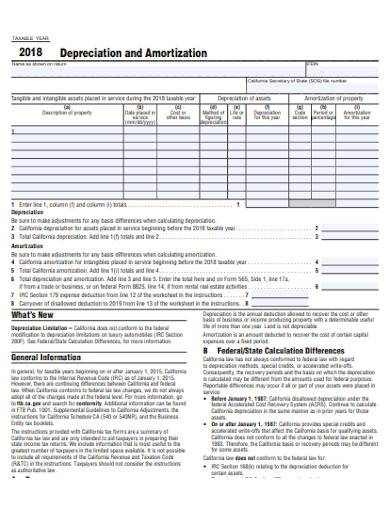ftb.ca.gov

## 7. Depreciating Assets Worksheet Template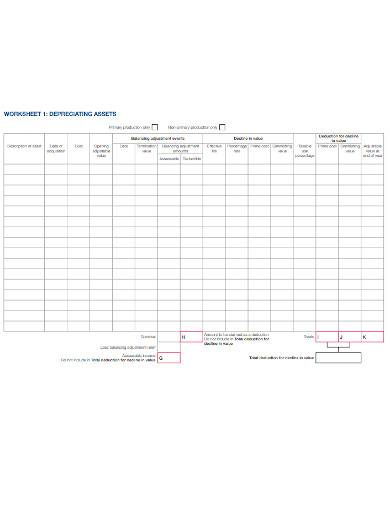ato.gov.au

## 8. Depreciation Methods Worksheet Template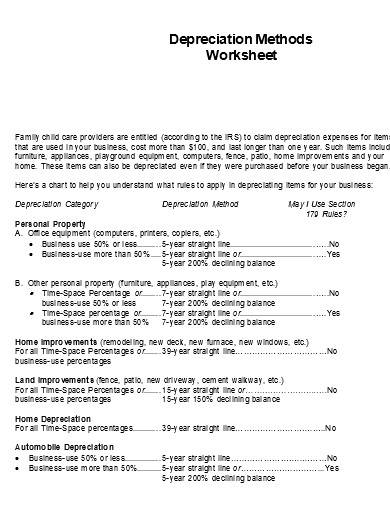firstchildrensfinance.org

## What is a Depreciation Worksheet?

A depreciation worksheet is an asset worksheet template that enables businesses to keep all their depreciation records stored in one centralized place, including their taxable gain, loss, or breakeven. Owning a business asset means that you have to keep track of its original basis or the cost of acquiring every asset and the depreciation you have claimed as years pass. With a depreciation schedule, you can track your long-term assets and determine how they will depreciate over time.

## How to Use a Depreciation Worksheet

Depreciation is a tool used as a part of accounting management plan to distribute the valuable asset’s costs over a certain number of an accounting period. The business utilizes a depreciation schedule instead of gathering the entire expense in one period. There are different methods that business owners can use to create and maintain their depreciation schedule management plan and each of these methods calculates a part of their expense which is recognized in their business financial statements.

### Step 1: Determine the Purchase Price of the Asset

The first step is determining the salvage value of your asset which is its expected sale value at the end of its useful life. You also have to determine the asset’s purchase price and its expected life in years.

### Step 2: Calculate the Asset’s Value

To calculate the value of your asset, take the cost of the asset and subtract it from the salvage value. Divide the resulting value by the total number of years it is expected to be useful. This will be used for assets that are expected to degrade at a stable rate over a period of time.

### Step 3: Calculate the Current Depreciation

Using the Units-for-Production method, you can calculate your asset’s current depreciation by dividing the expected units to be produced each year by the total expected units over its lifetime. Then multiply its result by the difference between the price and salvage value of the asset.

### Step 4: Use Double-Declining Method

Another method to use in determining your current depreciation is the double-declining method. Here, you divide two by the asset’s expected life span in years, then multiply the result by the estimated book value for every period.

## What are the different methods of depreciation?

The different methods of depreciation include the straight-line method, the double-declining balance method, the sum-of-the-years’-digits, and the units of production method.

## What is a depreciation schedule?

A depreciation schedule is a financial tool that enables companies to forecasted their fixed assets using balance sheet templates, depreciation expense or income statements, and capital expenditures or cash flow statements.

## What is the financial impact of depreciation?

Depreciation is considered by companies to be an expense with no related cash outflow. It is because companies have net cash outflows in the whole amount of the asset when it was first purchased. Therefore, no further cash-related activity will take place.

Company assets such as pieces of equipment and machinery are costly. Rather than computing the entire cost of an asset in a single year, business owners utilize depreciation to distribute the cost of their assets and match their depreciation expenses to other relevant revenues in the same period of time. This enables businesses to dismiss the value of an asset over a given time period.